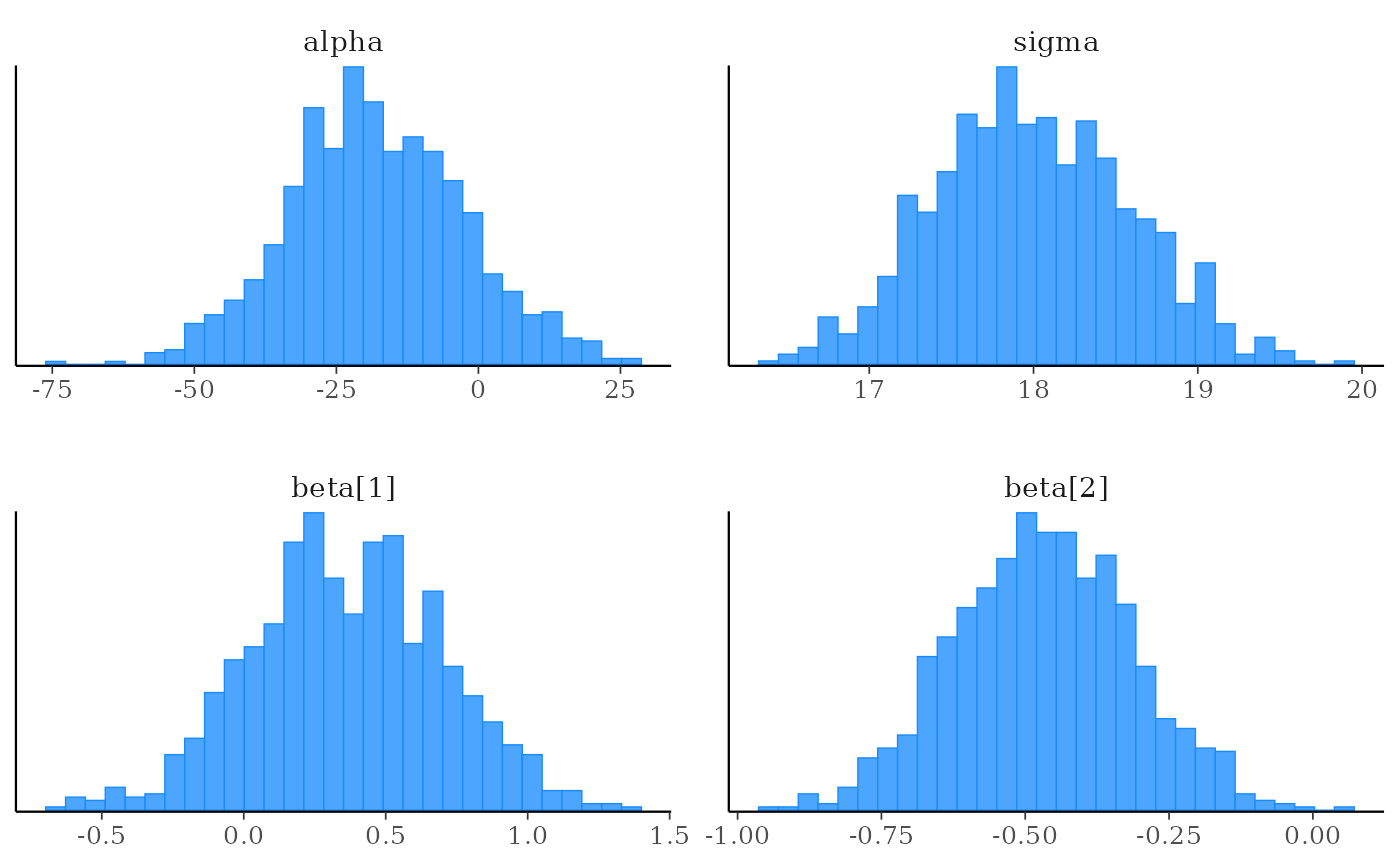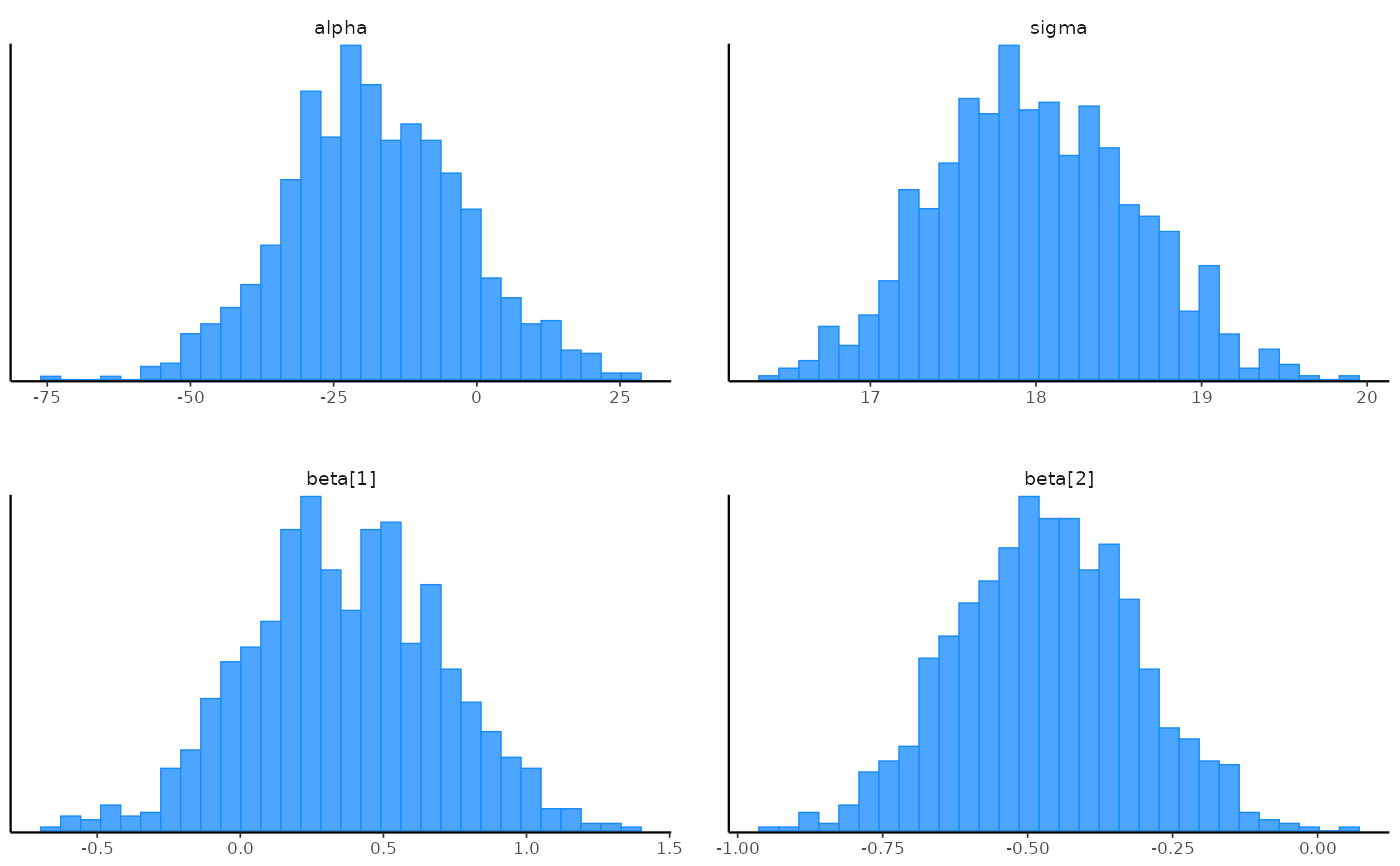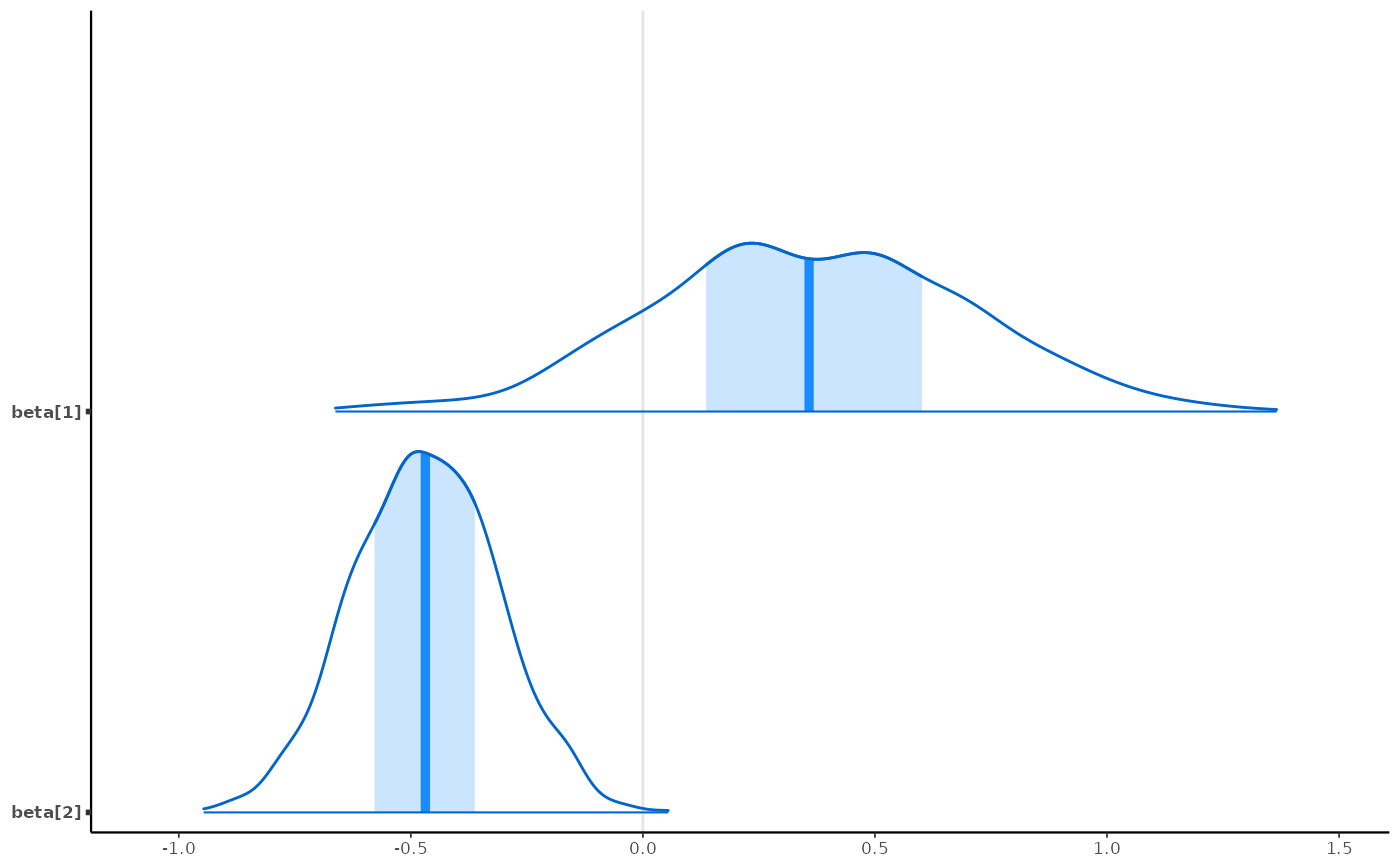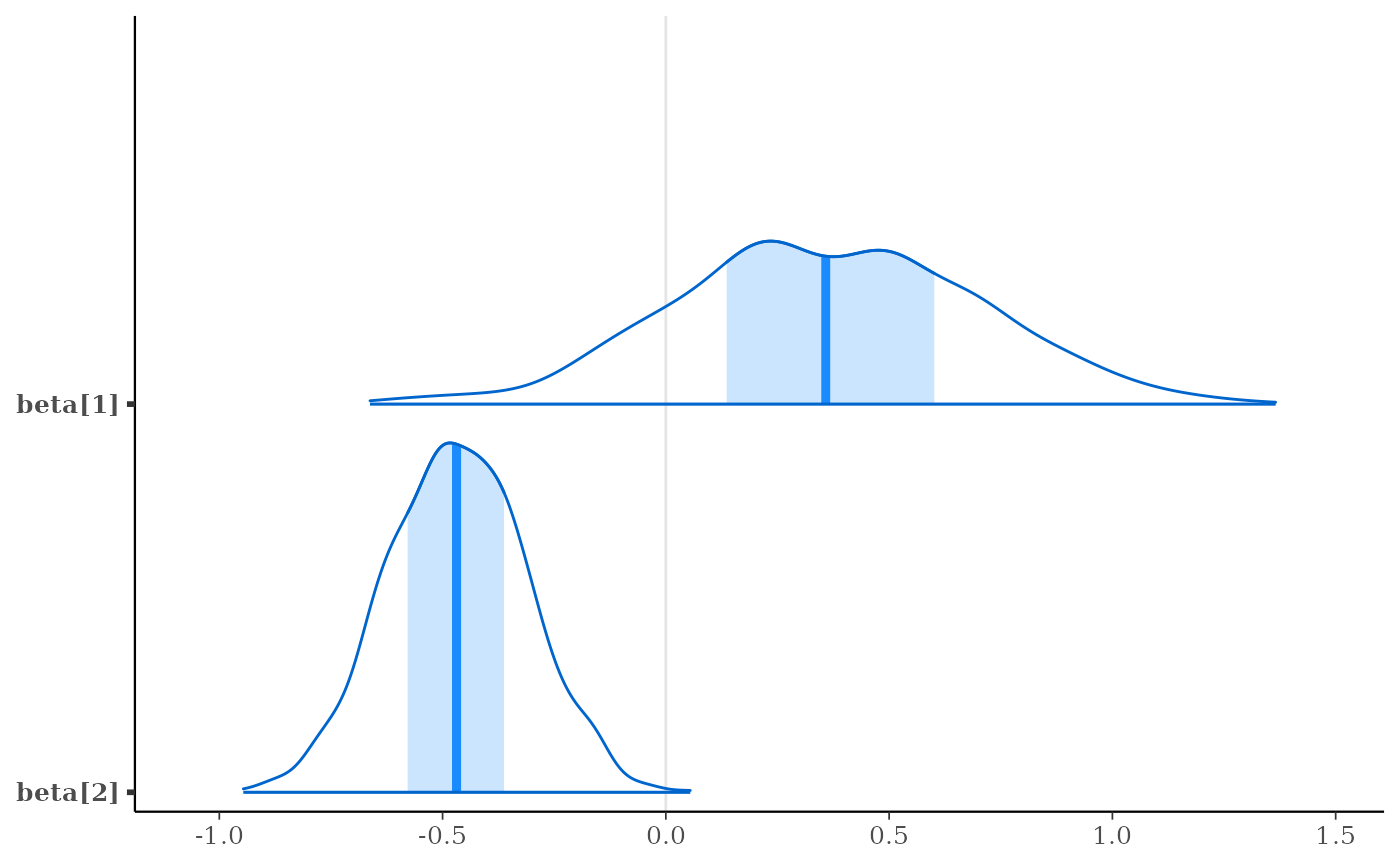The theme_default() function returns the default ggplot theme used by the bayesplot plotting functions. See bayesplot_theme_set() for details on setting and updating the plotting theme.

theme_default(
base_size = getOption("bayesplot.base_size", 12),
base_family = getOption("bayesplot.base_family", "serif")
)

## Arguments

base_size, base_family Base font size and family (passed to ggplot2::theme_bw()). It is possible to set "bayesplot.base_size" and "bayesplot.base_family" via options() to change the defaults, which are 12 and "serif", respectively.

## Value

A ggplot theme object.

## See also

bayesplot_theme_set() to change the ggplot theme.

bayesplot-colors to set or view the color scheme used for plotting.

bayesplot-helpers for a variety of convenience functions, many of which provide shortcuts for tweaking theme elements after creating a plot.

## Examples

class(theme_default())#>  "theme" "gg"
bayesplot_theme_set() # defaults to setting theme_default()
x <- example_mcmc_draws()
mcmc_hist(x)#> stat_bin() using bins = 30. Pick better value with binwidth.# change the default font size and family for bayesplots
bayesplot_theme_set(theme_default(base_size = 8, base_family = "sans"))
mcmc_hist(x)#> stat_bin() using bins = 30. Pick better value with binwidth.mcmc_areas(x, regex_pars = "beta")# change back
bayesplot_theme_set()
mcmc_areas(x, regex_pars = "beta")